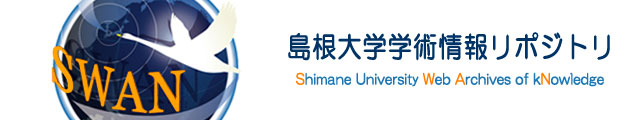ダウンロード数 : ?
 タイトルヨミ トクビョウ チョウサ ニオケル ケイザイテキ ヒョウホン チュウシュツ タンイ ノ カタ ニツイテ 日本語以外のタイトル On the Economical Unit of Sampling Survey for Seedlings in Nurseries ファイル 言語 日本語 著者 安井 鈞 藤田 直四郎 内容記述（抄録等） The type of unit affects usually both the accuracy and the cost of a sampling survey therefore, it may be veryinportant to find the optimum type of unitin the sampling survey of seed-bed. To find the economical unit type, the authors made some reserch works in the nursery of Simane Forest Experiment Station by the survey of Kuromatsu and Sugi seedlings. For a comparative study, we prepared eight type of unit, as shown in Table 1 and Fig. 1. Following the accurate population total number of seedlings was as certained in the areas by a census, we could get the variance of population per unit due to each types. Next were estimated the cost of sampling works by a time study (last row of Table 3 and 4). In studes of this type the item to be estimated is population total number of seedlings. If finite population correction is ignored, the variance of the estimated population total is(N^2_u S^2_u)/(n_u)Since this variance is to be the same for all units, the smallest unit (Type 1) was chosen as a standard. Then the values of the other n that give the same precision as the type I are obtained from above equation, as shown in the first lines of Table 7 and 8.Next step is to find the comparable sample size in term of type I. (second line of same Tables).The cost ot taking n of the type I may be expressed as (Kuromatsu)c_1 = (n_1)/(47.2)the values of the other c that compared with the type I are shown on the third lines of same Table.The type IV unit appers the best to be used for Kuromatsu and Sugi seedlings. The last lines of Table 7 and 8 shows the reciprocals of the costs compared with the Type I (0.1×0.1m) taken as 100. In the tables these figures have been called relative net precision. 掲載誌名 島根農科大学研究報告 巻 9 開始ページ (A-2)37-43 ISSN 05598311 発行日 1961-03-31 NCID AN00108241 出版者 島根農科大学 出版者別表記 The Shimane Agricultural College 資料タイプ 紀要論文 部局 生物資源科学部 備考 A,Bを含む 開学十周年記念号（Commemoration Number for the 10th Anniversary）